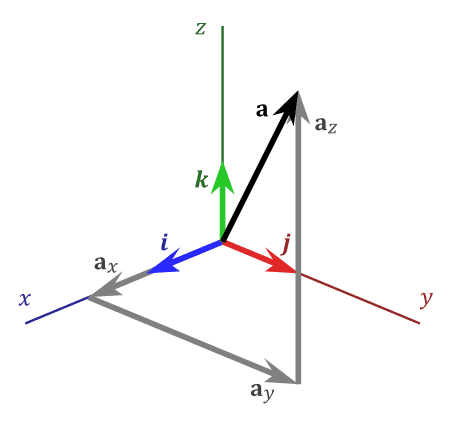# 3D coordinates vector calculation

• I
• runningc

#### runningc

How do I calculate the resultant of three component vectors set mutually at 60 degrees to each other (not in the same plane)?

Hi and welcome to PF.
The most straightforward way would be to use Cartesian Co-ordinates and resolve each of the three vectors along arbitrary x, y and z axes and add the x components together and likewise for the y and z components. Then the overall resultant vector is given by adding the three resulting components.

... Then the overall resultant vector is given by adding the three resulting components.

Where, of course, “adding the three resulting components” is vector addition. The resultant magnitude is found by adding the magnitudes in quadrature and the angular direction requires resolving to some angular representation, probably Euler angles, by appropriate trigonometry.

Frankly I’d just leave it as components. It’s easier, just as meaningful and twice as useful. (for those of us who favor linear algebra)

•sophiecentaur
Frankly I’d just leave it as components.
Agreed - unless you actually need to draw a line on a graph.

•Cutter Ketch
And the Wikipedia article is a pretty good introduction:

https://en.wikipedia.org/wiki/Euclidean_vector